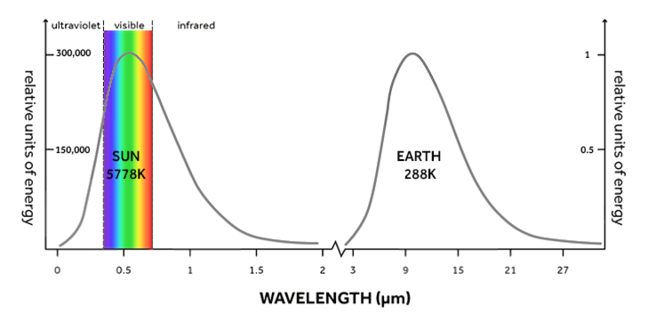3.2

# The Sun and the Earth

What determines the climate of the Earth? Ultimately, the Sun, our nearest star.

Solar energy is absorbed by the Earth’s surface, warming it and driving the weather patterns and therefore the climate that we observe. The only other source of energy at the Earth’s surface comes from the cooling of the interior of the planet, but this is about 2000 times smaller than the flux of energy we get from the Sun.

With a surface temperature of just over 5,500°C (or 5,778K, where K stands for Kelvin, the international unit of temperature) the Sun emits a lot of energy into space. The temperature determines both how much energy it emits, and what form it takes (Planck’s Law describes this). In the case of the Sun, most energy is light – some shorter wavelength ultra-violet and some longer wavelength infrared radiation (heat) as you can see in Figure 1. Because the surface of the Earth is much cooler, 15°C (or 288K), the Earth emits much less energy into space.

In the graph in Figure 1, the scale on the vertical, Y axis for the Earth goes up to 1 compared to 300,000 relative units of energy for the Sun. Also, the Earth only loses infrared radiation (heat) to space, which has a much longer wavelength than the infrared radiation the Sun emits. If you look at the horizontal, X axis (wavelength) you can see a gap between the emission curves of the Sun and the Earth. The two curves never overlap. This means that, as the Sun’s and the Earth’s energy pass through the atmosphere, very different processes can operate on the two.##### Figure 1: The energy output of the Sun (left) and the Earth (right). Both the amount of energy a body produces, and the wavelength of the energy (in this case, whether it is ultraviolet, light or heat) are determined by the temperature of the body.

In the next Step, we look at the relationship between the energy lost from the Sun and the Earth to space, in further detail.